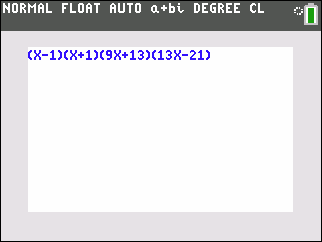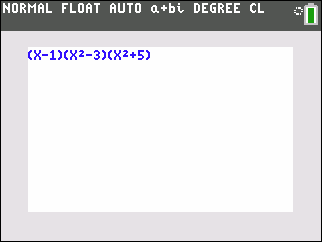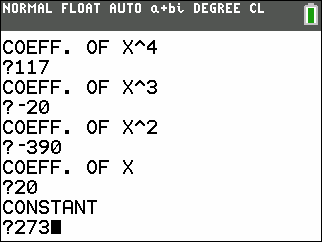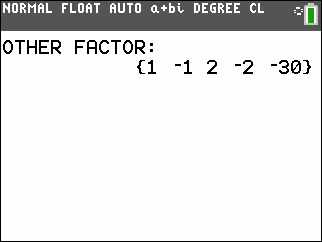Basics Archives Community Services ProgrammingHome :: Archives :: File Archives :: FACTORFACTOR

FILE INFORMATION

factor.zip
 Filename factor.zip (Download) Title FACTOR Description FACTOR: This program finds the linear factors of a polynomial of one variable. Try for example 117X^4 -20X^3 -390X^2 +20X +273. If the polynomial only has rational factors it will be completely factored. The above example will factor as (X-1)(X+1)(9X+13)(13X-21), If the polynomial has irrational or imaginary/complex roots in addition to rational roots, or if the program can't find any factor, the program will show the coefficients of the remaining polynomial. For example: X^3 +X^2 +X -3, will give a linear factor of (X-1), the program will then display: OTHER FACTOR: {1 2 3}. Which means that 1X^2 +2X +3, is another integral factor. If the program can't find any linear factor, or when there is not enough of them, it tries a substitution for X^2. It can then find factors like, (AX^2+B). This makes it more likely that it finds all the integral factors. Try for example: X^5-X^4+2X^3-2X^2-15X+15.The program is now three times faster for the first equation above, than the original version. FACTORS: This is the same program as FACTOR, except that if the equation will not allow itself to be factored at all, or partly, it ,or the rest of it, will be printed out on the graph screen. This comes at a cost of an additional 128 bytes. Authors Anders Tiberg (anders.tiberg@telia.com)Joe Erdeky (erdeky@inbox.com) Category TI-84 Plus C Silver Edition/CE BASIC Math Programs File Size 3,260 bytes File Date and Time Mon Sep 17 16:31:21 2018 Documentation Included? Yes

SCREEN SHOTSREVIEWS

There are no reviews for this file.

LEAVE FEEDBACK

Questions, comments, and problems regarding the file itself should be sent directly to the author(s) listed above.

Report inappropriate or miscategorized file (requires an account; or you may email us directly)